# Plot model coefficients with ggcoef_model()

library(ggstats)

The purpose of ggcoef_model() is to quickly plot the coefficients of a model. It is an updated and improved version of GGally::ggcoef() based on broom.helpers::tidy_plus_plus(). For displaying a nicely formatted table of the same models, look at gtsummary::tbl_regression().

## Quick coefficients plot

To work automatically, this function requires the {broom.helpers}. Simply call ggcoef_model() with a model object. It could be the result of stats::lm, stats::glm or any other model covered by {broom.helpers}.

data(tips, package = "reshape")
mod_simple <- lm(tip ~ day + time + total_bill, data = tips)
ggcoef_model(mod_simple)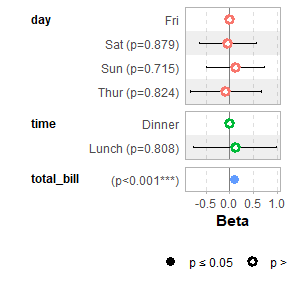In the case of a logistic regression (or any other model for which coefficients are usually exponentiated), simply indicated exponentiate = TRUE. Note that a logarithmic scale will be used for the x-axis.

d_titanic <- as.data.frame(Titanic)
d_titanic$Survived <- factor(d_titanic$Survived, c("No", "Yes"))
mod_titanic <- glm(
Survived ~ Sex * Age + Class,
weights = Freq,
data = d_titanic,
family = binomial
)
ggcoef_model(mod_titanic, exponentiate = TRUE)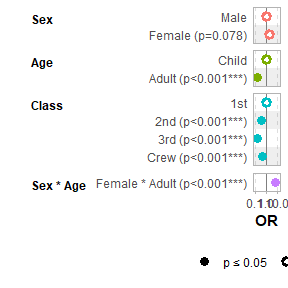## Customizing the plot

### Variable labels

You can use the {labelled} package to define variable labels. They will be automatically used by ggcoef_model(). Note that variable labels should be defined before computing the model.

library(labelled)
tips_labelled <- tips %>%
set_variable_labels(
day = "Day of the week",
time = "Lunch or Dinner",
total_bill = "Bill's total"
)
mod_labelled <- lm(tip ~ day + time + total_bill, data = tips_labelled)
ggcoef_model(mod_labelled)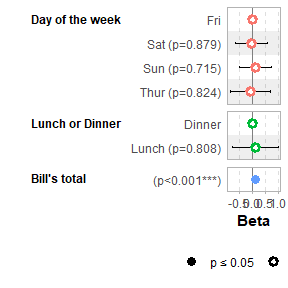You can also define custom variable labels directly by passing a named vector to the variable_labels option.

ggcoef_model(
mod_simple,
variable_labels = c(
day = "Week day",
time = "Time (lunch or dinner ?)",
total_bill = "Total of the bill"
)
)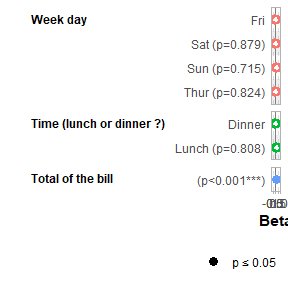If variable labels are to long, you can pass ggplot2::label_wrap_gen() or any other labeller function to facet_labeller.

ggcoef_model(
mod_simple,
variable_labels = c(
day = "Week day",
time = "Time (lunch or dinner ?)",
total_bill = "Total of the bill"
),
facet_labeller = ggplot2::label_wrap_gen(10)
)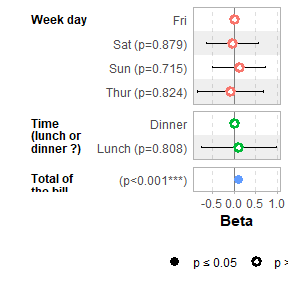Use facet_row = NULL to hide variable names.

ggcoef_model(mod_simple, facet_row = NULL, colour_guide = TRUE)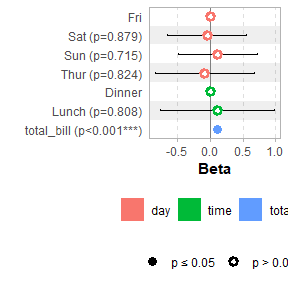### Term labels

Several options allows you to customize term labels.

ggcoef_model(mod_titanic, exponentiate = TRUE)ggcoef_model(
mod_titanic,
exponentiate = TRUE,
show_p_values = FALSE,
signif_stars = FALSE,
categorical_terms_pattern = "{level} (ref: {reference_level})",
interaction_sep = " x "
) +
ggplot2::scale_y_discrete(labels = scales::label_wrap(15))
#> Scale for y is already present.
#> Adding another scale for y, which will replace the existing scale.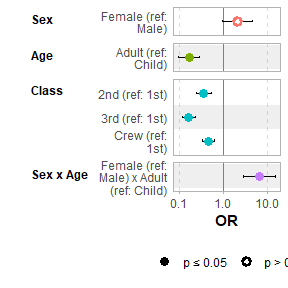By default, for categorical variables using treatment and sum contrasts, reference rows will be added and displayed on the graph.

mod_titanic2 <- glm(
Survived ~ Sex * Age + Class,
weights = Freq,
data = d_titanic,
family = binomial,
contrasts = list(Sex = contr.sum, Class = contr.treatment(4, base = 3))
)
ggcoef_model(mod_titanic2, exponentiate = TRUE)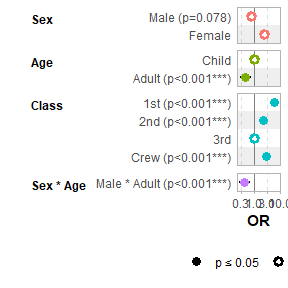Continuous variables with polynomial terms defined with stats::poly() are also properly managed.

mod_poly <- lm(Sepal.Length ~ poly(Petal.Width, 3) + Petal.Length, data = iris)
ggcoef_model(mod_poly)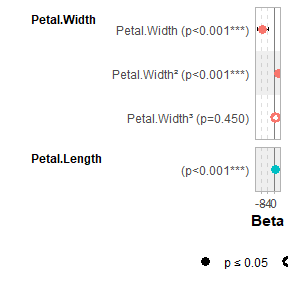Use no_reference_row to indicate which variables should not have a reference row added.

ggcoef_model(
mod_titanic2, exponentiate = TRUE,
no_reference_row = "Sex"
)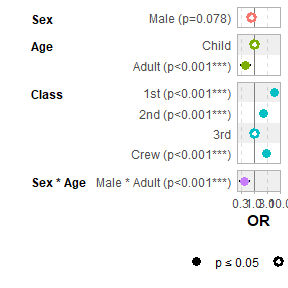ggcoef_model(
mod_titanic2, exponentiate = TRUE,
no_reference_row = broom.helpers::all_dichotomous()
)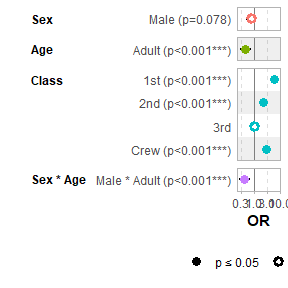ggcoef_model(
mod_titanic2, exponentiate = TRUE,
no_reference_row = broom.helpers::all_categorical(),
categorical_terms_pattern = "{level}/{reference_level}"
)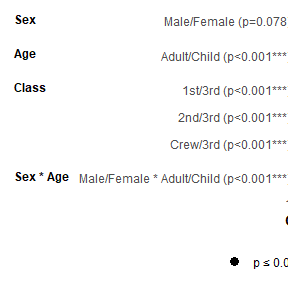### Elements to display

Use intercept = TRUE to display intercepts.

ggcoef_model(mod_simple, intercept = TRUE)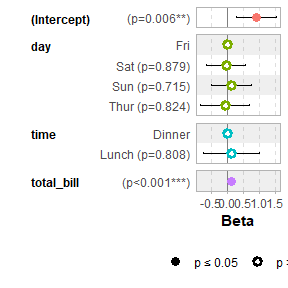You can remove confidence intervals with conf.int = FALSE.

ggcoef_model(mod_simple, conf.int = FALSE)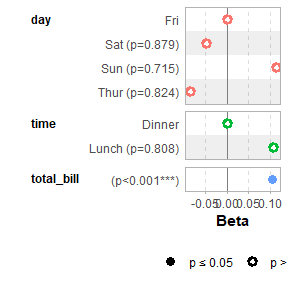By default, significant terms (i.e. with a p-value below 5%) are highlighted using two types of dots. You can control the level of significance with significance or remove it with significance = NULL.

ggcoef_model(mod_simple, significance = NULL)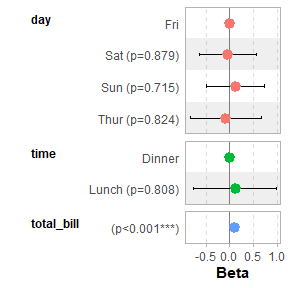By default, dots are colored by variable. You can deactivate this behavior with colour = NULL.

ggcoef_model(mod_simple, colour = NULL)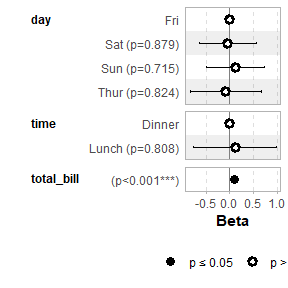You can display only a subset of terms with include.

ggcoef_model(mod_simple, include = c("time", "total_bill"))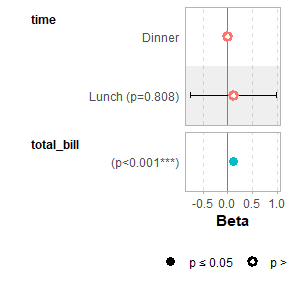It is possible to use tidyselect helpers.

ggcoef_model(mod_simple, include = dplyr::starts_with("t"))You can remove stripped rows with stripped_rows = FALSE.

ggcoef_model(mod_simple, stripped_rows = FALSE)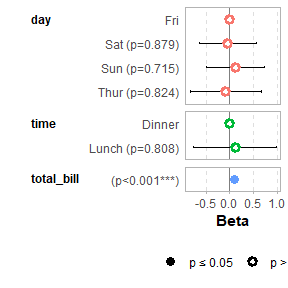Do not hesitate to consult the help file of ggcoef_model() to see all available options.

### ggplot2 elements

The plot returned by ggcoef_model() is a classic ggplot2 plot. You can therefore apply ggplot2 functions to it.

ggcoef_model(mod_simple) +
ggplot2::xlab("Coefficients") +
ggplot2::ggtitle("Custom title") +
ggplot2::scale_color_brewer(palette = "Set1") +
ggplot2::theme(legend.position = "right")
#> Scale for colour is already present.
#> Adding another scale for colour, which will replace the existing scale.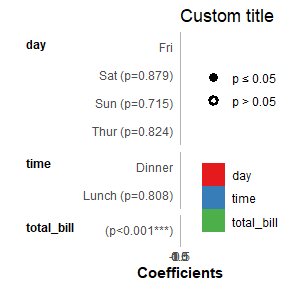## Multinomial models

For multinomial models, simply use ggcoef_multinom(). Two types of visualizations are available: "dodged" and "faceted".

library(nnet)
mod <- multinom(Species ~ ., data = iris)
#> # weights:  18 (10 variable)
#> initial  value 164.791843
#> iter  10 value 16.177348
#> iter  20 value 7.111438
#> iter  30 value 6.182999
#> iter  40 value 5.984028
#> iter  50 value 5.961278
#> iter  60 value 5.954900
#> iter  70 value 5.951851
#> iter  80 value 5.950343
#> iter  90 value 5.949904
#> iter 100 value 5.949867
#> final  value 5.949867
#> stopped after 100 iterations
ggcoef_multinom(
mod,
exponentiate = TRUE
)
#> ! broom::tidy() failed to tidy the model.
#> ✔ tidy_parameters() used instead.
#> ℹ Add tidy_fun = broom.helpers::tidy_parameters to quiet these messages.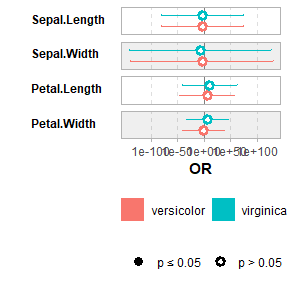ggcoef_multinom(
mod,
exponentiate = TRUE,
type = "faceted"
)
#> ! broom::tidy() failed to tidy the model.
#> ✔ tidy_parameters() used instead.
#> ℹ Add tidy_fun = broom.helpers::tidy_parameters to quiet these messages.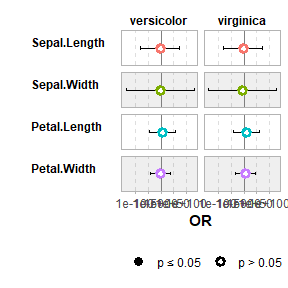ggcoef_multinom(
mod,
type = "faceted",
y.level_label = c("versicolor" = "versicolor\n(ref: setosa)")
)
#> ! broom::tidy() failed to tidy the model.
#> ✔ tidy_parameters() used instead.
#> ℹ Add tidy_fun = broom.helpers::tidy_parameters to quiet these messages.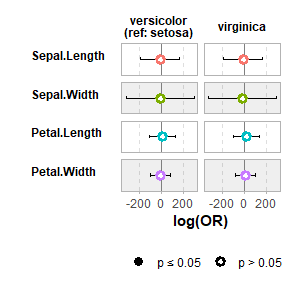## Comparing several models

You can easily compare several models with ggcoef_compare().

mod1 <- lm(Fertility ~ ., data = swiss)
mod2 <- step(mod1, trace = 0)
mod3 <- lm(Fertility ~ Agriculture + Education * Catholic, data = swiss)
models <- list(
"Full model" = mod1,
"Simplified model" = mod2,
"With interaction" = mod3
)

ggcoef_compare(models)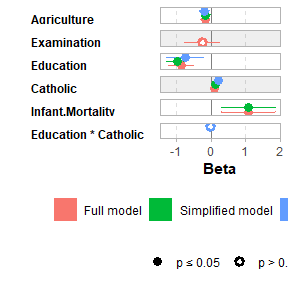ggcoef_compare(models, type = "faceted")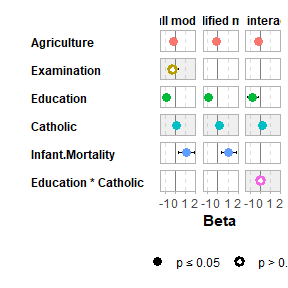Advanced users could use their own dataset and pass it to ggcoef_plot(). Such dataset could be produced by ggcoef_model(), ggcoef_compare() or ggcoef_multinom() with the option return_data = TRUE or by using broom::tidy() or broom.helpers::tidy_plus_plus().

## Supported models

model notes
biglm::bigglm()
biglmm::bigglm()
brms::brm() broom.mixed package required
cmprsk::crr() Limited support. It is recommended to use tidycmprsk::crr() instead.
fixest::feglm() May fail with R <= 4.0.
fixest::femlm() May fail with R <= 4.0.
fixest::feNmlm() May fail with R <= 4.0.
fixest::feols() May fail with R <= 4.0.
gam::gam()
geepack::geeglm()
glmmTMB::glmmTMB() broom.mixed package required
lavaan::lavaan() Limited support for categorical variables
lfe::felm()
lme4::glmer() broom.mixed package required
lme4::glmer.nb() broom.mixed package required
lme4::lmer() broom.mixed package required
logitr::logitr() Requires logitr >= 0.8.0
MASS::glm.nb()
MASS::polr()
mgcv::gam() Use default tidier broom::tidy() for smooth terms only, or gtsummary::tidy_gam() to include parametric terms
mice::mira Limited support. If mod is a mira object, use tidy_plus_plus(mod, tidy_fun = function(x, ...) mice::pool(x) %>% mice::tidy(...))
multgee::nomLORgee() Experimental support. Use tidy_multgee() as tidy_fun.
multgee::ordLORgee() Experimental support. Use tidy_multgee() as tidy_fun.
nnet::multinom()
ordinal::clm() Limited support for models with nominal predictors.
ordinal::clmm() Limited support for models with nominal predictors.
parsnip::model_fit Supported as long as the type of model and the engine is supported.
plm::plm()
rstanarm::stan_glm() broom.mixed package required
stats::aov() Reference rows are not relevant for such models.
stats::glm()
stats::lm()
stats::nls() Limited support
survey::svycoxph()
survey::svyglm()
survey::svyolr()
survival::clogit()
survival::coxph()
survival::survreg()
tidycmprsk::crr()
VGAM::vglm() Limited support. It is recommended to use tidy_parameters() as tidy_fun.

Note: this list of models has been tested. {broom.helpers}, and therefore ggcoef_model(), may or may not work properly or partially with other types of models.